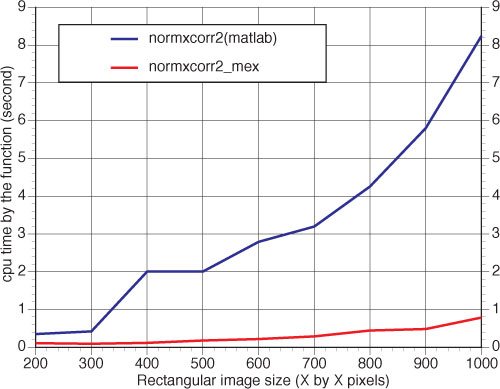## Update on the normxcorr2_mex comparison

I just found out that I didn’t do a fair enough comparison between the MATLAB built-in normxcorr2 function and the pre-compiled normxcorr2_mex in the previous post. The reason is that MATLAB is calculating the full normalized cross-correlation, while the pre-compiled MEX file only calculates the values for the “valid” region of the cross correlation. The idea of calculating only the “valid” region assumes that the feature/template that you are looking for is WITHIN the larger image. If the feature has several pixels sticking out of the larger image, this assumption will make the normxcorr_mex to give the wrong peak position.

So, to match the output of the normxcorr2 in matlab, one will need to pad the larger image with zeros before using the normxcorr2_mex. For example, instead of using
`ncc = normxcorr2_mex(template, img);`
The following code will give you exactly the same result as the output from the Matlab normxcorr2 function:
```img_padded=padarray(img,[size(template,1)-1,size(template,2)-1]); ncc=normxcorr2_mex(template, img_padded, 'valid');```

By doing this, we are calculating more correlation values, thus trading off performance, as is indicated by the comparison plot below (still 10 times speed-up with large image sizes, compared with the matlab built-in function) :### 2 Responses to “Update on the normxcorr2_mex comparison”

1. Jame Delashmit Says:

Thanks… amazing discussion. Uncover on-line about the particular tips.

2. transactional funding Says:

Hi there Dear, are you in fact visiting this website regularly, if so afterward you will absolutely take nice
knowledge.